# 「CS231n Course 3」神经网络简介

Posted by saltyfishyjk on 2023-05-24
Words 3k and Reading Time 11 Minutes
Viewed Times

# 「CS231n Course 3」神经网络简介

## Part 1 反向传播

### 从（标量）简单表达式引入

（偏）导数的意义是，当函数变量在某个点周围的极小区域内变化，而导数是变量变化导致的函数在该方向上的变化率，数学定义如下：

### 使用链式法则计算复合表达式

#### 计算图例子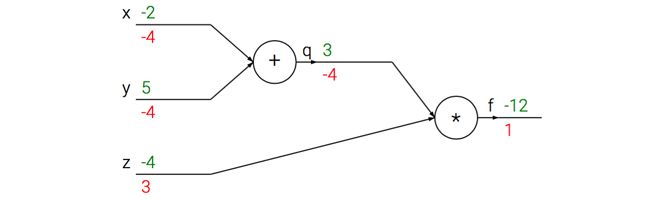• 前向传播（forward pass）：绿色数字是量的值（ $x,y,z$ 的初始值是我们设定的，没有特殊含义），从左到右（前向）进行依次通过算子（节点（node）/门（gate））

• 反向传播（backword pass）：红色数字是 $f$ 相对于当前变量（假定为 $u$ ）的梯度，从右到左（反向/后向）依次通过算子进行链式法则。$f$ 的梯度初始值是 $1$，这是因为 $\frac{\partial f}{\partial f} = 1$

#### 直观理解

• 前向传播计算：输入是若干节点的值，输出是经过该节点的运算结果。如上图中$+$门的两个输入是 $-2$ 和 $5$，输出是 $-2+5=3$
• 反向传播计算：输入是链式法则上一步的梯度，输出是链式法则的下一步梯度。如上图中$\times$门的输入是 $1$，输出有两个分支方向，对于 $q$ 方向，由于 $\times$ 运算的偏导数是对方（如$f(x, y) = xy \quad \frac{\partial f}{\partial x} = y \quad \frac{\partial f}{\partial y} = x$），因此局部梯度为 $z$ 即为 $-4$，因此链式法则的下一步梯度得到 $1 \times (-4) = -4$；对于 $z$ 方向，由于 $\times$ 运算的偏导数是对方，因此局部梯度为 $q$ 即为$3$，因此链式法则的下一步梯度得到 $1 \times 3 = 3$

### 模块化：以 $Sigmoid$ 为例

#### 计算图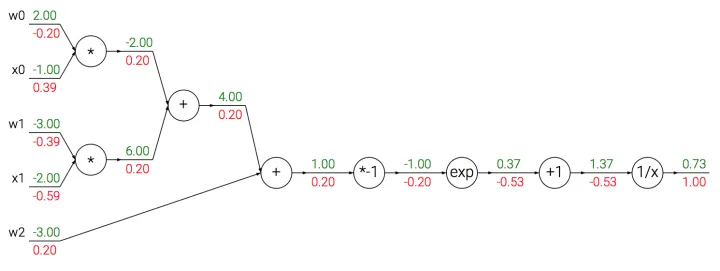#### $Sigmoid$

$Sigmoid$ 函数也被记为 $\sigma (x)$，形式如下：

### 回传流中的模式

#### $max$ 门

$max$ 门将梯度转给其中一个输入，该输入是前向传播中值最大的那个输入。

## Part 2 神经网络

### 神经元（Neuron）

#### 连接与信号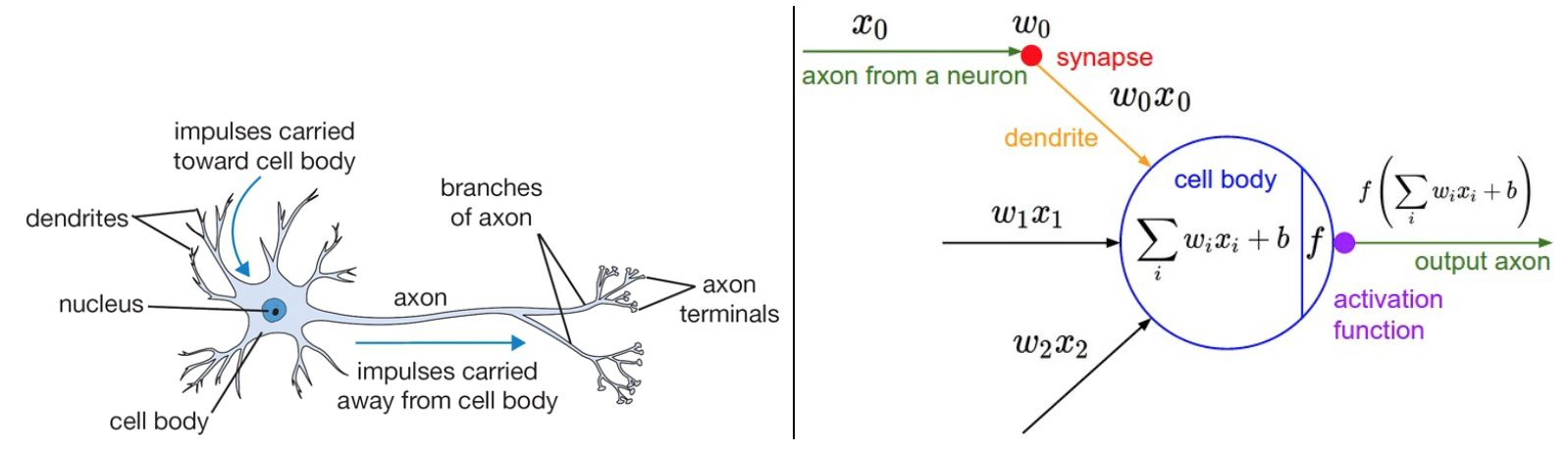• 生物模型中，每个神经元从树突获得输入信号，沿着唯一轴突产生输出信号。轴突在末端会分支，通过突触和其他神经元的树突相连。
• 计算模型中，沿着轴突传播的信号（这里是 $x_0$），传播至下一个神经元时，会基于突触的强度（这里是 $w_0$），和该神经元的树突进行乘法交互（这里是 $w_0x_0$）。这里，突触的强度（也就是权重 $w$ ）是可以学习的，而且可以控制一个神经元对另一个神经元影响的强度（还可以控制影响方向：使其兴奋（正权重）或抑制（负权重））
• 生物模型中，树突将信号传递到细胞体，信号在细胞体中相累加，如果和高于某个阈值，则神经元被激活，向其轴突输出一个峰值信号。
• 计算模型中，假设峰值信号的准确时间点不重要，重要的是激活信号的频率。这个基于速率编码（firing rate）的观点将神经元的激活率建模为激活函数（activation function）$f$ ，表达轴突上的激活信号的频率。
• 历史上激活函数常选择使用 sigmoid 函数 $\sigma$，该函数输入实数值（求和后的信号值），将输入值压缩到 0-1 之间。

### 常用激活函数

#### $Sigmoid$

$Sigmoid$ 公式如下：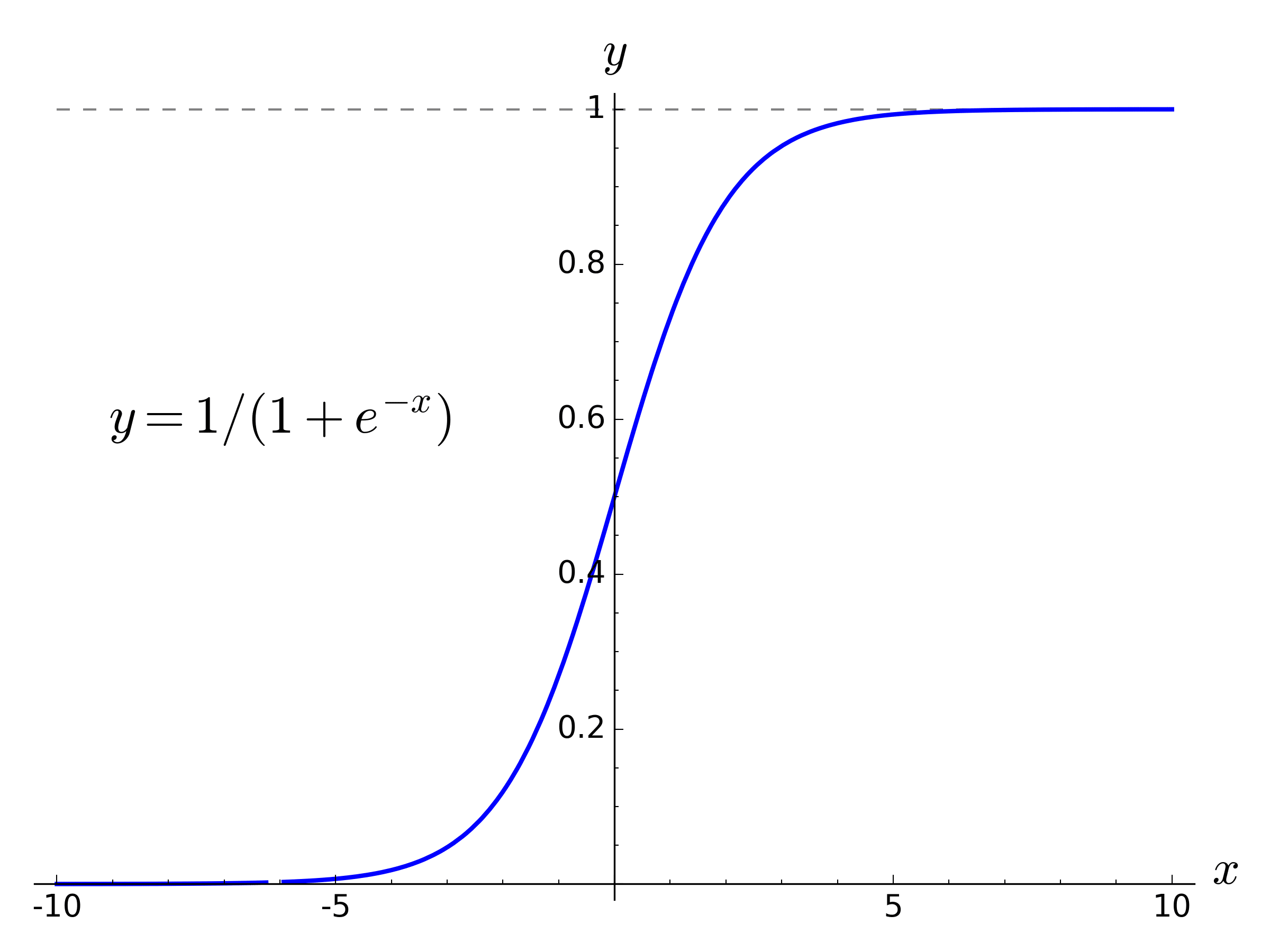$Sigmoid$ 函数输入实数值，并将其压缩到 $0$ 到 $1$ 的范围内。具体地，很大的负数变为 $0$ ，很大的正数变为 $1$ 。

$Sigmoid$ 对于神经元的激活频率有良好的解释：从完全不激活（$0$）到在求和后的最大频率处的完全饱和的激活（$1$）。尽管历史上比较常用，但是现在已经很少使用了，因为有两个主要缺点：

• $Sigmoid$ 函数饱和使梯度消失。当神经元的激活在接近 $0$ 或 $1$ 处时会饱和，梯度接近 $0$。由于反向传播时，局部梯度会和输出的梯度相乘，如果局部梯度很小（接近 $0$ ），那么相乘的结果会接近 $0$ ，这回“杀死”梯度。
• $Sigmoid$ 函数的输出不是零中心的。

#### $tanh$

$tanh$ 公式如下：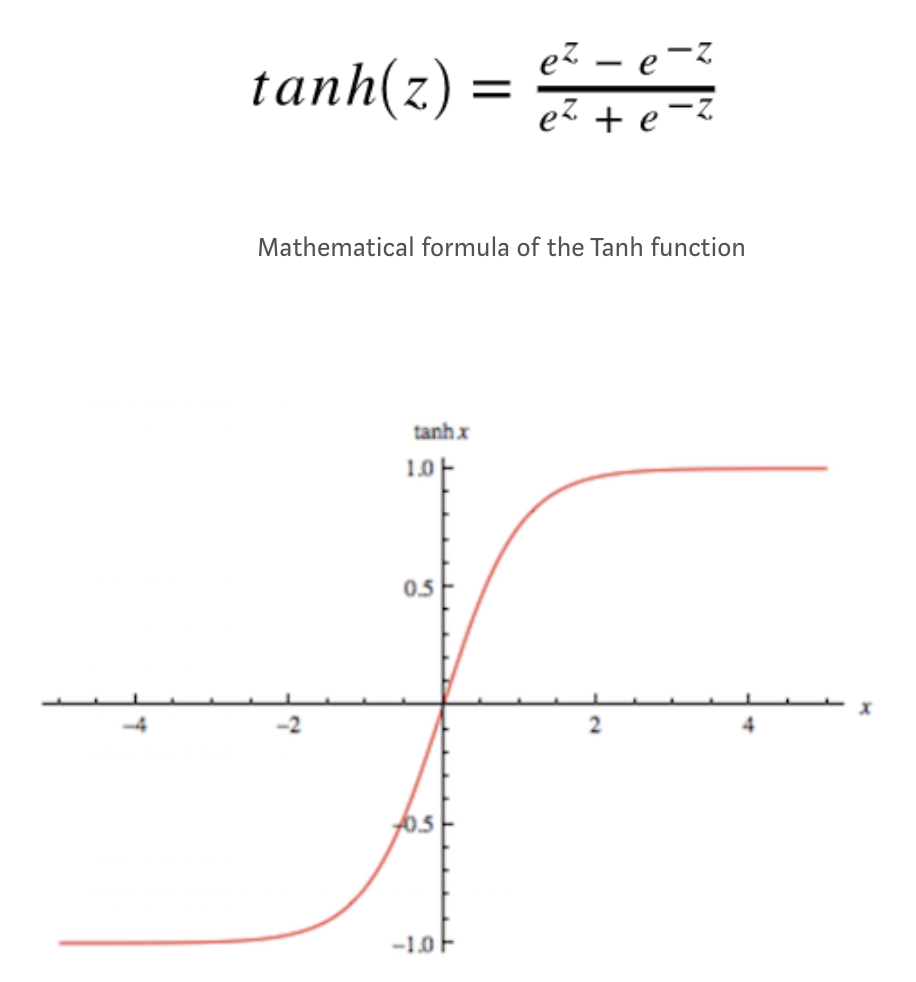ReLU公式如下：

TODO

Leakly ReLU公式如下：

TODO

Maxout公式如下：

TODO

### 神经网络结构

#### 分层组织（layer-wise organization）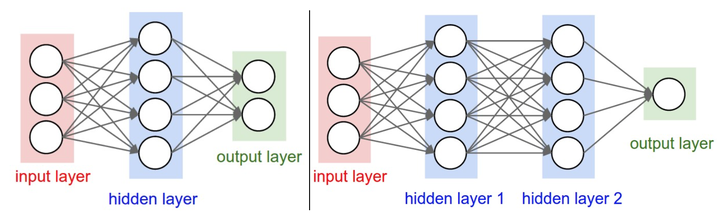#### 命名规则

##### 确定网络尺寸（Sizing neural networks）

• 左侧网络有 $4+2=6$ 个神经元（输入层不算），$[3 \times 4] + [4 \times 2]=20$ 个 权重（weights），$4+2=6$ 个偏置（bias），共 $26$ 个可学习的参数。

• 右侧网络有 $4+4+2=10$ 个神经元（输入层不算），$[3 \times 4]+[4 \times 4]+[4 \times 1]=32$ 个权重（weights）， $4+4+1=9$ 个偏置（bias），共 $41$ 个可学习的参数。

#### 前向传播计算举例（feed-forward computation）